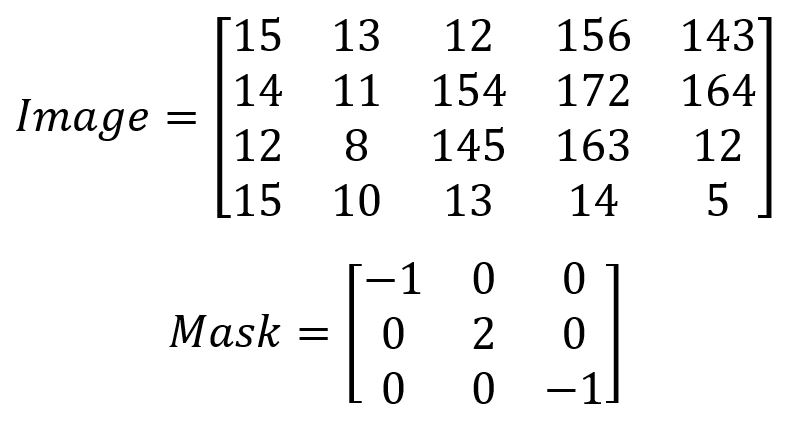Robotics 2
Checkpoint 2: Vision and AIExample Question 2:  In an 'Object Matrix', what does the value of each pixel represent? If we start from an RGB image, what are the steps to get to an object matrix?
Example Question 3:  AI problem solving and searching algorithms are categorized in two different ways.  What are the two categorizations?  Write a short description of each category.
Example Question 4:  For the categories you defined in Question 3, why might we choose to use an algorithm in one category, rather than another?  For each category, give one example of a situation where an algorithm in that category would work better
Example Question 5: Some machine learning algorithms are 'supervised' algorithms, and some are 'unsupervised'.  What is the difference between these?  In what situations might we choose to use an algorithm in one rather than another?  Give one example of an algorithm that is an unsupervised learning algorithm, and one example of a supervised algorithm.
Example Question 7: In class, we learned about two supervised learning algorithms.  What were these two algorithms?  Briefly explain how each of these algorithms works.  What pros and cons does each algorithm have?  In what case might we choose to use one rather than the other?
Example Question 8:  In class, we learned about two methods for detecting motion.  What were these two methods?  Give some pros and cons for each method, and briefly explain how each method works.
Example Question 1: Suppose I have the image and mask as shown here.  Find the result image.
Example Question 9:  Is it true that it is better to use more features rather than fewer features?  Why or why not?  How could you determine when you have selected the right number of features?
Example Question 10:  List and briefly explain the three rules for selecting which features to use in a machine learning algorithm for machine vision.
Congratulations!  You've made it all the way through our second unit: Vision and AI.  Before we move on, you will need to take a test to make sure that all this information has sunk in.  Here are some example problems like the ones you will see on the test in class.  On our review day (the class day before the test), I'll go through these problems live on the board.  So, it's a good idea to try to solve the problems on your own before coming to class.Example Question 6:  What does it mean for data to be 'separable'?  Draw one picture that illustrates data that IS separable, and one picture that illustrates data that IS NOT separable.  Why do we  care about the separability of data when we are doing machine learning?